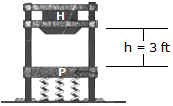# Engineering Mechanics - KOP: Impulse and Momentum - Discussion

### Discussion :: KOP: Impulse and Momentum - General Questions (Q.No.2)

2.The drop hammer H has a weight of 900 lb and falls from rest. H has a weight of 900 lb and falls from rest h = 3 ft onto a forged anvil plate P that has a weight of 500 lb. The plate is mounted on a set of springs which have a combined stiffness of kT = 500 lb/ft. Determine (a) the velocity of P and H just after collision and (b) the maximum compression in the springs caused by the impact. The coefficient of restitution between the hammer and the plate is e = 0.6. Neglect friction along the vertical guide posts A and B.

 [A]. vH2 = 3.97 ft/s, vP2 = 17.87 ft/s, x = 4.15 ft [B]. vH2 = 8.94 ft/s, vP2 = 8.94 ft/s, x = 2.57 ft [C]. vH2 = 5.96 ft/s, vP2 = 14.30 ft/s, x = 3.52 ft [D]. vH2 = 20.8 ft/s, vP2 = 12.51 ft/s, x = 3.20 ft

Explanation:

No answer description available for this question.